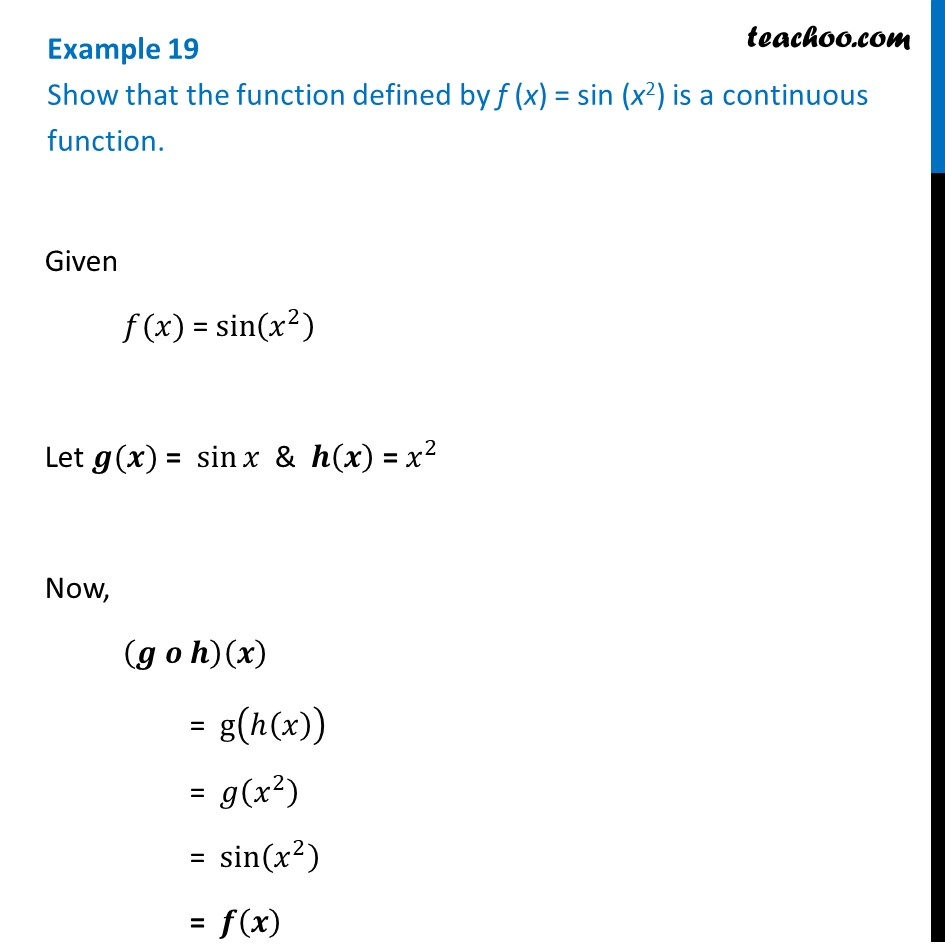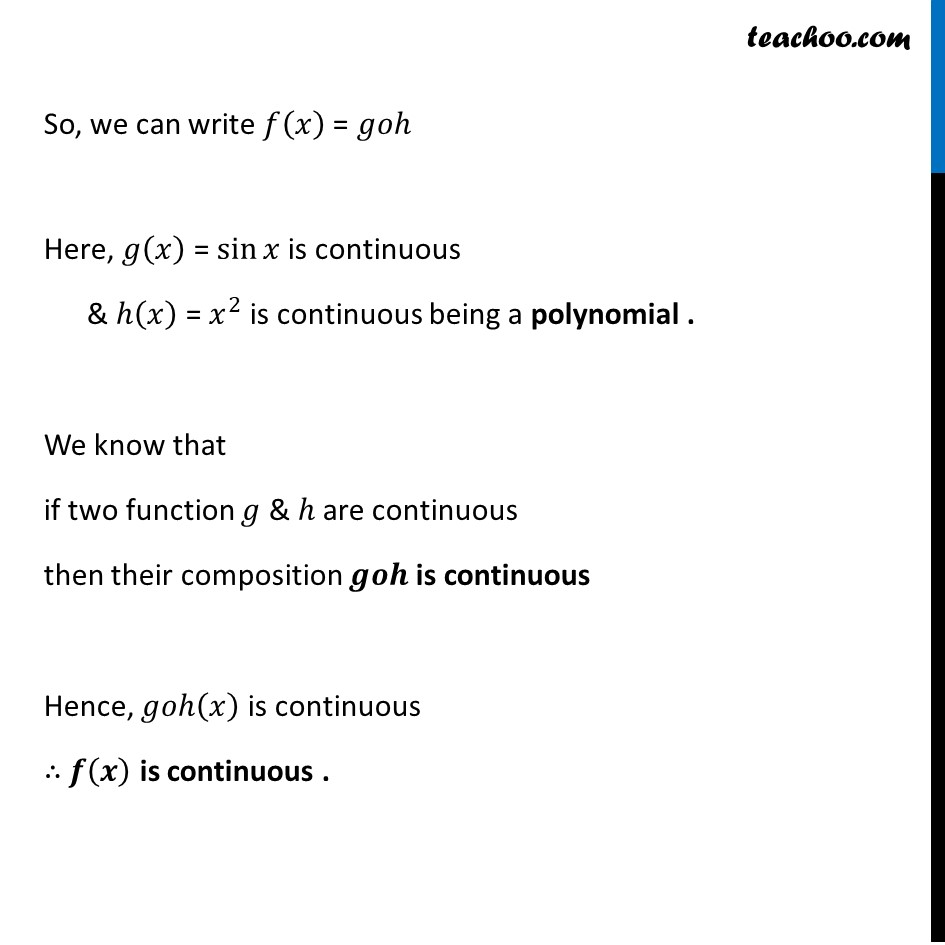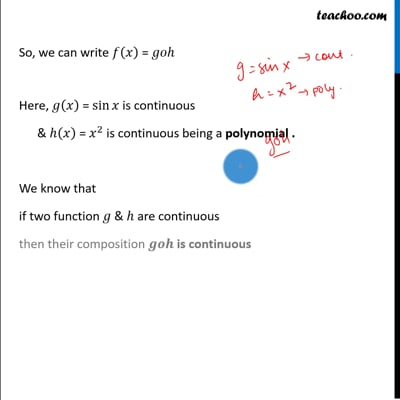Continuity of composite functions

Chapter 5 Class 12 Continuity and Differentiability
Concept wiseThis video is only available for Teachoo black users

Introducing your new favourite teacher - Teachoo Black, at only ₹83 per month

### Transcript

Example 19 Show that the function defined by f (x) = sin (x2) is a continuous function.Given 𝑓(𝑥) = sin⁡(𝑥^2 ) Let 𝒈(𝒙) = sin⁡𝑥 & 𝒉(𝒙) = 𝑥^2 Now, (𝒈 𝒐 𝒉)(𝒙) = g(ℎ(𝑥)) = 𝑔(𝑥^2 ) = sin⁡(𝑥^2 ) = 𝒇(𝒙) So, we can write 𝑓(𝑥) = 𝑔𝑜ℎ Here, 𝑔(𝑥) = sin⁡𝑥 is continuous & ℎ(𝑥) = 𝑥^2 is continuous being a polynomial . We know that if two function 𝑔 & ℎ are continuous then their composition 𝒈𝒐𝒉 is continuous Hence, 𝑔𝑜ℎ(𝑥) is continuous ∴ 𝒇(𝒙) is continuous .